# Numerical Modeling of the Effects of Fiber Packing and Reinforcement Volume Ratio on the Transverse Elasticity Modulus of a Unidirectional Composite Material Glass / Epoxy

Numerical Modeling of the Effects of Fiber Packing and Reinforcement Volume Ratio on the Transverse Elasticity Modulus of a Unidirectional Composite Material Glass / Epoxy
Mechanical Engineering Department, ABBES Laghrour University, Khenchela 40004, Algeria

Corresponding Author Email:
brek_samir@univ-khenchela.dz
Page:
203-210
|
DOI:
https://doi.org/10.18280/rcma.305-602
Received:
3 August 2020
|
Accepted:
6 December 2020
|
Published:
30 December 2020
| Citation

OPEN ACCESS

Abstract:

Composite materials are very widely used in the manufacturing of structures because of their specific mechanical properties. However, they are characterized by heterogeneity and anisotropy and they present great challenges in designing and also in predicting their behavior by using the numerical simulation. The unidirectional composite material has a more relevant property which is the transverse elasticity modulus E2. The determination of E2 is still interesting researchers because of the diversity of results obtained by several models and approaches. This study aims to predict the transverse elasticity modulus E2 of a unidirectional Glass/Epoxy composite material, the effect of the arrangement fibers on the transverse elasticity modulus and predict the values of the reinforcement factor used in the Halpin-Tsai model. To do so first we adopted the micromechanical approach, which is accurate but requires much computing, and we used a calculation code based on FEM method and considered two parameters to vary, which are the volume fraction of fibers and the distribution of fibers. The obtained results of numerical modeling were tightly compared to those obtained by the available analytical models and the adopted approach can be used to predict the transverse elasticity modulus E2 and the reinforcement factor $\xi$.

Keywords:

micromechanics analysis, prediction of properties, reinforcing factor, transverse modulus, unidirectional lamina

1. Introduction

Today, composite materials are more and more used in the realization of structures in many fields such as transport, automobile, shipbuilding, sports accessories and more particularly aeronautics and aerospace because of their high performance/mass ratio compared to other more conventional materials such as steel or aluminum and also the possibility of obtaining complex shapes by reducing the number of leads, this is due to the use of materials with specific mechanical characteristics such as carbon, glass or Kevlar and the use of the production processes layer by layer with molds and counter molds [1-4].

Unidirectional composites are those that have all fibers aligned in one direction, their mechanical properties vary with the orientations of the fibers therefore these materials are anisotropic. The strength of unidirectional composites in the direction of the fibers is generally dominated by the properties of the fibers while in the transverse direction it is dominated by the properties of the matrix. However, the heterogeneity and anisotropy of composite materials present great challenges to the design of structures, consequently to the numerical simulation of their behavior. In order to respond to these challenges, the current trend is multi-scale modeling because it makes it possible to predict the effective response of heterogeneous materials from their microstructure, and it even gives the possibility of designing new materials with desired or optimized properties. There are two basic approaches are usually considered in the modeling of composites: the macromechanical approach and the micromechanical one.

In the macromechanical approach, the composite is considered as an anisotropic material and the details of the arrangement of the constituent materials are ignored and the micromechanical one aims to develop solutions to be able to predict the effective behavior of a heterogeneous anisotropic material depending on the behavior of the constituent materials and their arrangement . Most theories of homogenization are limited to a few idealized mathematical models of two-phase systems. Many analytical approaches have been developed over the years [6-8], and comprehensive bibliographic studies have been published on the prediction of longitudinal and transverse elastic modulus of unidirectional composites, the estimation of the reinforcement factor $\xi$ and the use of the model of Halpin-Tsai to determine the transverse elasticity modulus E2 [9-18].

The analytical methods provide a reasonable prediction for relatively simple configurations of the phases for example square or triangular arrangements of the fibers but when it is about complex geometries numerical methods are used for approximate solutions by simplifying hypotheses on microstructures and boundary conditions, as it is the case with finite element method. Numerical methods for calculating the properties of composite materials generally involve the analysis of a representative volume element (RVE).

This work aims mainly to predict the transverse elasticity modulus of a unidirectional Glass/Epoxy composite material with different values of fiber volume fractions, to study the effect of the arrangement randomize of the fibers on the values of the transverse elastic modulus and to predict the values of the reinforcement factor $\xi$.

In section 2 we introduced the analytical models based on the mixture rule, these models will be used to compare our obtained results.

Section 3 is the main part of this work, it is devoted to numerical modeling, in this section we used Cast3m as a calculation software based on finite element method, the numerical model was developed by using the homogenization method which is a micromechanical approach. The analysis is performed on a representative volume element (RVE).

Finally, the obtained results are compared to those obtained by different models based on the representative elementary volume.

2. Analytical Models Based on the Rule of Mixture

The analytical method uses various mathematical expressions to predict elastic constants such as the stiffness and strength of the composite material. An example of the different methods used to assess the elastic properties of the composite material, for example the rule of mixture method, the Halpin-Tsai model, the Nielsen method and the Chamis model.

2.1 Rule of mixture (ROM)

This is the simplest method to determine the elastic properties of a unidirectional composite material. The classical ROM accurately predicts the longitudinal Young's modulus E1, Eq. (1), Poisson's ratio v12, Eq. (2), but does not accurately predict the transverse Young's modulus, in general and more particularly when the fiber volume is high, Eq. (3) [19-21].

$E_{1}=E_{f L} \cdot V_{f}+E_{m} \cdot\left(1-V_{f}\right)($Voigt model$)$       (1)

$v_{12}=v_{f} \cdot V_{f}+v_{m} \cdot\left(1-V_{f}\right) \quad($Voigt model$)$           (2)

$E_{2}=\frac{E_{f t} \cdot E_{m}}{E_{f t} \cdot\left(1-V_{f}\right)+E_{m} \cdot V_{f}} \quad($Reuss model$)$        (3)

where, EfL, Eft, vf are fiber properties (longitudinal elastic modulus, transversal elastic modulus and Poisson’s ratio, respectively), Em, vm are matrix properties (elastic modulus and Poisson’s ratio, respectively) and Vf is the fiber volume fraction.

2.2 Halpin-Tsai (HT) and modified Halpin-Tsai (HTM) models

The Halpin-Tsai equation, Eq. (4), was developed as a semi-empirical model in order to produce more complex results on the transverse Young's modulus and the longitudinal shear modulus .

$E_{2}=E_{m}\left(\frac{1+\xi \cdot \eta \cdot V_{f}}{1-\eta \cdot V_{f}}\right)$      (4)

The coefficient $\eta$ is given by: $\eta=\frac{\left(\frac{E f t}{E_{m}}\right)-1}{\left(\frac{E_{f t}}{E m}\right)+\xi}$.

$\xi$ is an empirical factor, which measures the reinforcement of the fibers of the composite material which depends on the geometry of the fiber, the arrangement of the fibers and the loading conditions. In generally, $\xi$ may vary from zero to infinity, and the Reuss and Voigt models, Eqns. (3) and (1) are special case for $\xi$=0 and $\xi \rightarrow \infty$, respectively.

Limiting values of $\eta$ are : for very rigid inclusions $\eta$=1, for homogeneous material $\eta$=0 and for voids $\eta=\frac{-1}{\xi}$ .

JC Halpin and SW Tsai obtain an excellent agreement with the results obtained by DF Adams and DR Doner, for the transverse modulus of a square array of circular fibers having a fiber volume fraction of 0.55, by a finite difference method applied to the case of cylindrical fibers distributed according to a square arrangement and for a volume fraction of fibers of 0.55, taking $\xi$=2 to calculate E2 and $\xi$=1 to calculate the shear modulus G12 [22, 23].

A general recommendation to be used when there is not experimental data for calibration is presented in the sequence, Eq. (5) .

$\xi=2+40 . V_{f}^{10}$ for $E_{2}$      (5)

Recently, a modified Halpin-Tsai model was proposed by Giner et al. , wherein a set of finite element analyses were performed considering randomly distributed unidirectional fibers for different volume fractions Vf and the following analytical expressions have been adjusted to the  $\xi$ estimations for random fiber arrangement, Eq. (6):

$\xi=\left\{\begin{array}{c}4.924-35.888 \mathrm{~V}_{f}+125.118 \mathrm{~V}_{f}^{2}-145.121 \mathrm{~V}_{f}^{3} \text { if } \mathrm{V}_{f}<0.3 \\ 1.5+5500 . \mathrm{V}_{\mathrm{f}}^{18} \text { if } \mathrm{V}_{f} \geq 0.3\end{array}\right.$         (6)

In some cases, for the reinforcement efficiency, a constant value of $\xi$ can be calculated from the result of the experimental test, or by numerical modeling with known volume fractions Vf and Vm. When reinforcement efficiency is higher, it means that the fibers contribute to the strength of the composite. The Halpin-Tsai method offers the advantage of being simple (easy to use in the design process) and offers a more precise prediction, the choice of the value of $\xi$ base limits of the use of this equation for a generalized case.

Neilson  modified the Halpin-Tsai equation by including the maximum fiber volume content $\emptyset_{\max }$ and the equation Eq. (4) transformed into Eq. (7):

$E_{2}=E_{m} \frac{\left(1+\xi \cdot \eta \cdot V_{f}\right)}{\left(1-\eta \cdot \psi \cdot V_{f}\right)}$         (7)

where, $\eta=\frac{\left(\frac{E}{E} t\right)-1}{\left(\frac{E t}{E m}\right)+\xi}$ and $\psi=1+\frac{\left(1-\phi_{\max }\right)}{\phi_{\max }^{2}} V_{f}$.

where, $\phi_{\max }$ is given by 0.785 for the square array of fibers, 0.907 for the hexagonal arrangement and 0.82 for random packing .

2.3 Chamis (CH) model

In the rule of mixture approach, for the evolution of the transverse modulus, it is assumed that the whole of the matrix and of the fiber are in series and therefore undergo the same transverse stress. In reality, we cannot consider that the whole matrix is in series with the fiber. To solve this problem, Hopkins and Chamis worked with a special RVE (fiber surrounded by the matrix), then they a determined the value of the transverse Young modulus E2 in both cases with and without interphase, for the case without interphase we have the formula Eq. (8) :

$E_{2}=\frac{E_{m}}{1-\left(\sqrt{V_{f}}\left(1-\frac{E_{m}}{E_{f t}}\right)\right)}$        (8)

2.4 Tsai with contiguity model

During the manufacture of fibrous composite materials, the fibers are often in a random arrangement rather than being in an ordinary arrangement, this randomness is much more typical for fibers of small diameter. Thus, the analysis concerning the modules of composite materials with regular arrangements must be modified to take into account the fact that the fibers are contiguous. Contiguity was introduced by Tsai as a method of making sense out of experimental data in comparison to theoretical predictions, and is based on fiber spacing and arrangement. In this case the fibers touching rather to be completely surrounded by the matrix. If c represents the degree of contiguity in the formula Eq. (9), then c = 0 corresponds to no contiguity (isolated fibers) and c = 1 corresponds to perfect contiguity (all the fibers are in contact). Naturally with volume fractions important fibers, c should approach c = 1. This approach is an example of what Chamis and Sendeckyj present as a semi-empirical method, but it could also be classified as a bound technique .

$E_{2}=A^{*}\left[(1-c) B^{*}+c . C^{*}\right]$       (9)

where, the constants A*, B* and C*:

$A^{*}=2\left[1-v_{f}+\left(v_{f}-v_{m}\right) \cdot V_{m}\right]$

$B^{*}=\frac{K_{f}\left(2 \cdot K_{m}+G_{m}\right)-G_{m}\left(K_{f}-K_{m}\right) V_{m}}{\left(2 \cdot K_{m}+G_{m}\right)+2\left(K_{f}-K_{m}\right) V_{m}}$

$C^{*}=\frac{K_{f}\left(2 \cdot K_{m}+G_{f}\right)+G_{f}\left(K_{m}-K_{f}\right) V_{m}}{\left(2 \cdot K_{m}+G_{f}\right)-2\left(K_{m}-K_{f}\right) V_{m}}$

$K_{f}=\frac{E_{f t}}{2\left(1-v_{f}\right)}, K_{m}=\frac{E_{m}}{2\left(1-v_{m}\right)}$

$G_{f}=\frac{E_{f t}}{2\left(1+v_{f}\right)}, G_{m}=\frac{E_{m}}{2\left(1+v_{m}\right)}$

Vm is the matrix volume fraction and $0 \leq c \leq 1$.

3. Finite Element Modeling

3.1 Objective

Numerical modeling is a reliable tool despite the fact that the time devoted to defining the geometric dimensions and the calculation time always represent a major disadvantage compared to analytical models. To study the influence of the random arrangement of long fibers on the values of the transverse elastic modulus E2 in a representative volume element (part of the unidirectional fold requested in transverse traction) and to predict the values of the reinforcement factor $\xi$. The numerical modelizations by finite elements are carried out using software Cast3m . We developed calculation programs (language GIBIANE). To expand the study two parameters were to be varied: The first is the position of the fibers by considering 50 cases so 50 representative elementary volumes (RVEs) were obtained, Figure 1. For each of 50 RVEs, 4 cases of the value of the radius of the fiber considered therefore 4 approximate values of volume fraction (44.3%, 48.8%, 53.6% and 58.6%), for example RVEs (n °1 and n°34), Figure 2. The effect of the voids content has not been taken into consideration in this work.

## 1.pngFigure 1. The models of RVEs for Vf = 0.443

## 2.png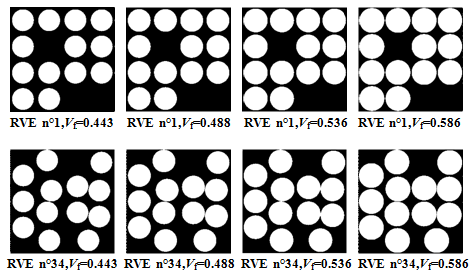Figure 2. RVEs (n °1 and n°34) for 44.3%,48.8%,53.6 % and 58.6% reinforcement volume ratio

3.2 Materials and characterizations

The composite material used in the numerical modeling corresponds to a unidirectional fold based on an epoxy resin and long E-glass fiber with circular section. Epoxy resin is a thermosetting polymer widely used in industry; it has excellent adhesion to glass fibers. Some mechanical properties of fiber glass and epoxy resin are summarized in Table 1.

Table 1. The elastic characteristics 

 The elastic characteristics E-Glass fiber Epoxy Density [Kg/m3] 2600 1200 Young’s modulus [GPa] 73 3.45 Shear modulus [GPa] 29.9 1.33 Poisson’s ratio 0.22 0.30 Fracture stress [MPa] 3400 70 Ultimate elongation [%] 4.5 2

3.3 Representative volume element (RVE)

The concept of representative volume element is the first to use numerical approximations of the effective properties of a composite. Square or cubic RVEs are used for most numerical approximations due to the ease of numerical resolution of limit value problems with these geometries. The difficulties encountered in generating statistical information on the distributions and the concentrations of particles cause difficulties for the rigorous determination of the sizes of RVE. Therefore, for most applications, the sizes of the RVEs have been rather arbitrary. In this work, we considered a square RVE model of side 48 $\mu \mathrm{m}$. Figure 3 shows a typical RVE. Each RVE is made up of 16 cells (fiber with matrix or matrix without fiber).

## 3.pngFigure 3. Cross section in plane 2-3 and sketch of the domain analyzed numerically

The boundary conditions listed are indicated in Table 2. Note that V and W are displacements according to directions 2 and 3 respectively, AB, CB, CD and DA indicate the sides of the model RVE, Figure 3. The axial load is modeled by a traction displacement acting along axis 2. For such loading conditions, the limits (DA and AB) of the RVE also correspond to lines of symmetry. The displacement $\delta$ applied to the finite element model to determine E2 causes a longitudinal deformation in direction 2, $\varepsilon_{22}=\frac{\delta}{L}$ .

The boundary conditions based on the lines (or face) of symmetry in the RVE are used in several research works, for example [9, 12, 14].

Table 2. Boundary conditions along the 2 and 3 directions

 Direction along axis 2 along axis 3 The side DA CB AB DC Displacement V=0 W free V=$\delta$ W free W=0 V free V and W free

3.4 Cell geometry used for meshes

Figure 4 contains the geometries of the cells used to make the meshes. Each cell is used for a subroutine with the possibility of rotation of the cell according to the angles 90°, 180° and 270°, for the realization of 50 RVEs. All the fibers are assumed to have the same diameter, and because of the complexity of the phenomenon and that the epoxy resin has excellent adhesion to glass fibers, the interface between the matrix and the fibers has not been taken into consideration in this work.

## 4.png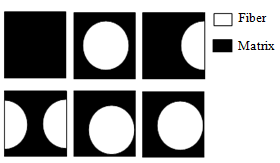Figure 4. The cells used to make the meshes of the RVEs

3.5 Mesh of the representative volume

In most of the finite element calculation softwares, the geometric model is created in two stages: first, the definition of the geometry by basic geometric elements then secondly the generation of the mesh from the created geometries. A mesh is the spatial discretization of a continuous medium with finite elements to solve the requested problem, the mesh of geometry constitutes an essential and most determining part of a numerical study. A good mesh is the mesh which allows having results close to reality and allows the calculation to rotate with the available means. Indeed, the finite element method consists in interpolating the value of the functions inside the meshes, therefore the larger the mesh, the greater the difference between the “real” value of the function and its interpolated value, therefore to have a result close to reality, we are often tempted to use a fine mesh .

The triangular element (Tri3) used for the realization of the meshes in the present analysis is based on a general state of 2D. The element comprises three nodes with two degrees of freedom per node. For example, RVE n °35 is composed of 859816 elements, Figure 5, same number of elements for RVE n °41, Figure 6.

## 5.png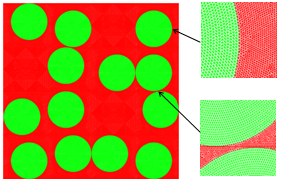Figure 5. Meshed model of RVE n °35, Vf = 0.443

## 6.png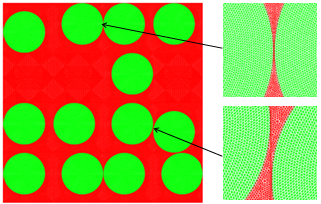Figure 6. Meshed model of RVE n °41, Vf = 0.443

3.6 Calculation of the transverse elasticity module E2

By assuming a linear elastic behavior, the generalized Hooke’s law in terms of the compliance matrix [S] for an orthotropic lamina is [21, 27]:

$\left\{\begin{array}{l}\varepsilon_{11} \\ \varepsilon_{22} \\ \varepsilon_{33} \\ \gamma_{23} \\ \gamma_{31} \\ \gamma_{12}\end{array}\right\}=\left(\begin{array}{cccccc}S_{11} & S_{12} & S_{13} & 0 & 0 & 0 \\ S_{12} & S_{22} & S_{23} & 0 & 0 & 0 \\ S_{13} & S_{23} & S_{33} & 0 & 0 & 0 \\ 0 & 0 & 0 & S_{44} & 0 & 0 \\ 0 & 0 & 0 & 0 & S_{55} & 0 \\ 0 & 0 & 0 & 0 & 0 & S_{66}\end{array}\right)\left\{\begin{array}{l}\sigma_{11} \\ \sigma_{22} \\ \sigma_{33} \\ \tau_{23} \\ \tau_{31} \\ \tau_{12}\end{array}\right\} .$              (10)

where, $\mathrm{S}_{11}=\frac{1}{E_{1}}, \mathrm{~S}_{22}=\frac{1}{E_{2}}, \mathrm{~S}_{33}=\frac{1}{E_{3}}, \mathrm{~S}_{12}=\frac{-v_{12}}{E_{1}}, \mathrm{~S}_{13}=\frac{-v_{13}}{E_{1}}, \mathrm{~S}_{23}=\frac{-v_{23}}{E_{2}}, \mathrm{~S}_{44}=\frac{1}{G_{23}}, \mathrm{~S}_{55}=\frac{1}{G_{13}}$ and $\mathrm{S}_{66}=\frac{1}{G_{12}}$.

As only a uniform strain in direction 2 is applied, the global equilibrium that $\sigma_{33}=0$ and $\tau_{23}=0$ due to the symmetry of the solution. Additionally, the plane strain condition implies that $\varepsilon_{11}=0, \gamma_{31}=0$ and $\gamma_{12}=0$. An explicit expression for elastic modulus E2, Eq. (11) :

$E_{2}=\frac{E_{1} \cdot \sigma_{22}}{E_{1} \cdot \varepsilon_{22}+v_{12}^{2} \cdot \sigma_{2.2}}$        (11)

Eq. (11) is used to estimate the elastic modulus E2 from numerical modeling, since $\varepsilon_{22}$ is the uniform deformation applied and the stress $\sigma_{22}$ is calculated by our program as the sum of the forces of reaction to the right limit divided by the section of the RVE (we have assumed a unit thickness). On the other hand, E1 and $v_{12}$ are obtained from the rule of mixtures, Eq. (1) and Eq. (2).

3.7 Calculation of the reinforcement factor $\xi$

To determine the reinforcement factor $\xi$, the value of the Young's modulus E2 is first calculated numerically using Eq. (11) and follows introduced into the formula of Halpin-Tsai, Eq. (4), this method is used in several research works, Eq. (12).

$\xi=\frac{E_{\mathrm{ft}}\left(E_{2}-E_{\mathrm{m}}\right)-\mathrm{V}_{\mathrm{f}} E_{2}\left(E_{\mathrm{ft}}-E_{\mathrm{m}}\right)}{E_{\mathrm{m}}\left[\left(E_{\mathrm{ft}}-E_{2}\right)-\mathrm{V}_{\mathrm{m}}\left(E_{\mathrm{ft}}-E_{\mathrm{m}}\right)\right]}$         (12)

4. Results and Discussion

4.1 The effect of the position of the fibers on the transverse modulus of elasticity E2

Figures 7 to 10 contains the results obtained from the modeling of the ratio E2/Emfor the 50 RVEs, in the interval of the reinforcement volume ratio considered. The results highlight the influence of the reinforcement rate and the arrangement of the long fibers on the overall mechanical behavior of the unidirectional Glass/Epoxy material and especially on the value of the transverse elastic modulus E2, for example the results of the RVE n °42 and higher than RVE n °5 of 19.77% for Vf = 0.443 and 38.89% for Vf = 0.586.

The results of RVEs n °3 and 28 show that the presence of a zone of resin without fiber in a direction perpendicular to the stresses $\left(\sigma_{22}\right)$ create a remarkable weakness for the zone of RVE therefore decreases the modulus of elasticity E2, for example results of RVE n °1 are higher than RVE n °3 by 3.38% for Vf = 0.443 and 9.90% for Vf = 0.586. Results of RVE n °2 are higher than RVE n °28 by 12.12 % for Vf = 0.443 and 32.73% for Vf = 0.586.

The random fiber distribution in certain cases gives a Young's modulus greater than the square or triangular distribution when there is a matrix zone without fibers, for example in the two cases:

1-results of RVE n °41 is higher than RVE n °1 of 9.80% for Vf = 0.443 and 15.05% for Vf = 0.586.

2-results of RVE n °36 is higher than RVE n °11 by 11.58% for Vf = 0.443 and 16.63% for Vf = 0.586.

The results highlight the influence of the radius of the E-glass fiber on the values of the transverse elastic modulus E2 of the unidirectional material because the value of the radius influences the overall random distribution of the fibers.

## 7.png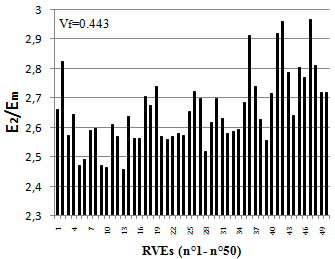Figure 7. Comparison between ratio E2/Em obtained for Vf = 0.443

## 8.png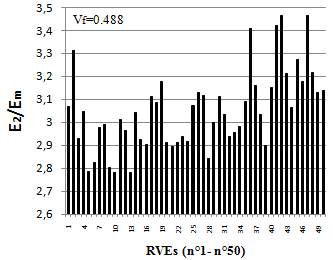Figure 8. Comparison between ratio E2/Em obtained for Vf = 0.488

## 9.png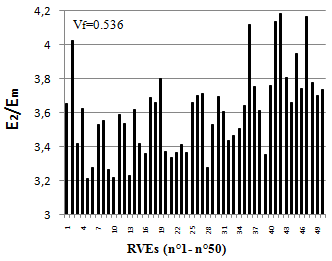Figure 9. Comparison between ratio E2/Em obtained for Vf = 0.536

## 10.pngFigure 10. Comparison between ratio E2/Emobtained for Vf = 0.586

4.2 Normal stress distribution $\sigma_{22}$

Figures 11a, 11b, 11c and 11d present, as an example, the normal stress distribution $\sigma_{22}$ in RVEs n°9 and n°34 for 0.443 reinforcement volume ratio. Figure 12, as an example, where there can be the areas of stress concentration between the fibers in the matrix area (RVE n°41).

## 11.pngFigure 11. Stress $\sigma_{22}$ distribution

## 12.pngFigure 12. Normal stress $\sigma_{22}$ distribution in RVEn °41, Vf =0.443

4.3 Transverse elasticity module as a function of the volume content of the fibers

Figure 13 shows the variation of the ratio E2/Em as a function of the volume fraction of the fibers in the interval considered and a comparison between the results obtained by numerical modeling and the values calculated using the analytical models ROM, Halpin-Tsai (with $\xi$=2), Chamis and Tsai with contiguity (with c=0.2 and c=0.5).

## 13.png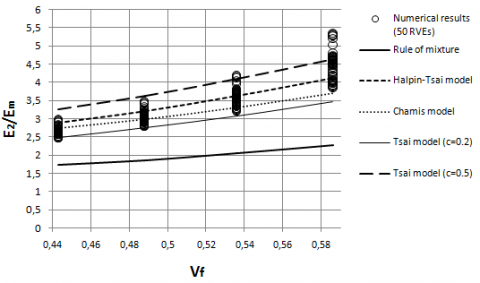Figure 13. Comparison between the modeling results and the values calculated by using analytical models

We can notice that the model of Chamis, Halpin-Tsai and Tsai with contiguity give much more agreement forecasts in the estimation of E2 on the contrary no RVE gives agreement for the calculation with the Rule of mixture (ROM).

For long fiber unidirectional Glass/Epoxy composites with a fiber volume content of less than 0.5, the Chamis and Halpin-Tsai model estimates the value of the transverse modulus better than the Tsai with contiguity (c=0.5) and ROM model. For Vf higher than 0.5 the Halpin-Tsai model ($\xi$=2) estimate the value of the transverse modulus better than the Tsai model with contiguity (c=0.2).

Using the results, we can draw the trend curve, Figure 14, suggest intervals, Eq. (13), or an approximate formula (coefficient of determination R2=0.876), Eq. (14), to estimate the value of the ratio E2/Em.

For $\frac{E_{f t}}{E_{m}}$,

$\left.\begin{array}{ll}2.4576 \leq \frac{E_{2}}{E_{m}} \leq 2.9677 & \mathrm{ifV}_{f}=0.443 \\ 2.7816 \leq \frac{E_{2}}{E_{m}} \leq 3.4638 & \mathrm{ifV}_{f}=0.488 \\ 3.2112 \leq \frac{E_{2}}{E_{m}} \leq 4.1846 & \mathrm{ifV}_{f}=0.536 \\ 3.8421 \leq \frac{E_{2}}{E_{m}} \leq 5.3366 & \mathrm{ifV}_{f}=0.586\end{array}\right\}$             (13)

If $0.443 \leq V_{f} \leq 0.586$,

$\frac{E_{2}}{E_{m}}=C_{1} \cdot V_{f}^{3}+C_{2} \cdot V_{f}^{2}+C_{3} \cdot \mid V_{f}+C_{4}$          (14)

where, the constants C1, C2, C3 and C4:

C1=202.3, $C_{2}=-267.4, C_{3}=126.1$ and $C_{4}=-18.32$.

## 14.png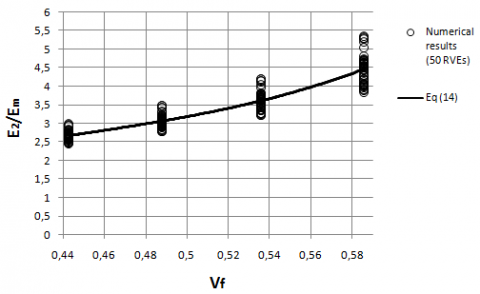Figure 14. Trend curve obtained by numerical models of the ratio E2/Em

## 15.png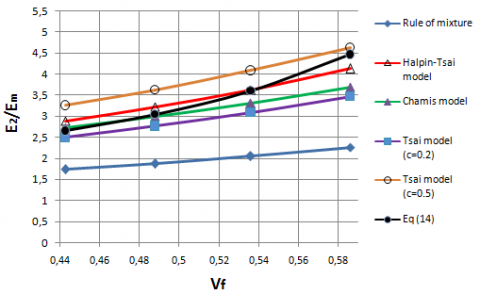Figure 15. Comparison between the trend curve and the values calculated using the analytical models

Figure 15 presents a comparison between the values calculated using analytical models and the results obtained by modeling which are represented by the trend curve, Eq. (14). For a value of Vf between 0.443 and 0.488 we can observe an agreement of the results obtained with the Chamis model, for the interval (between 0.53 and 0.55) and an agreement of the results with the Halpin -Tsai model with $\xi$= 2.

4.4 Reinforcement factor as a function of the volume content of the fibers

Figure 16 shows the variation of the reinforcement factor $\xi$ as a function of the volume fraction of the fibers and a comparison between the results obtained by the 50 RVEs and the values calculated using Eq. (5) and Eq. (6). We can suggest intervals, Eq. (15), or an approximate formula (coefficient of determination R2=0.451), Eq. (16), to estimate the value of the reinforcement factor $\xi$.

## 16.png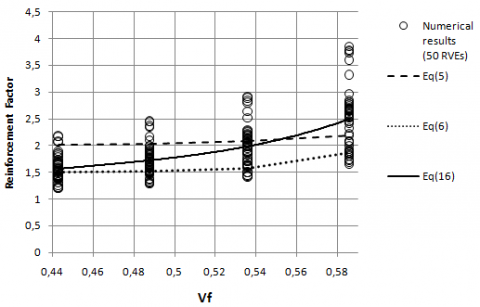Figure 16. The evolution of the reinforcement factor $\xi$ as a function of the volume fraction of the fibers

For $\frac{E_{f t}}{E_{m}}=21.1594$,

$\left.\begin{array}{ll}1.1901 \leq \xi \leq 2.1732 & \text { if } V_{f}=0.443 \\ 1.2825 \leq \xi \leq 2.4486 & \text { if } V_{f}=0.488 \\ 1.4066 \leq \xi \leq 2.9088 & \text { if } V_{f}=0.536 \\ 1.6440 \leq \xi \leq 3.8407 & \text { if } V_{f}=0.586\end{array}\right\}$                   (15)

If $0.443 \leq V_{f} \leq 0.586$,

$\xi=C_{1} \cdot V_{f}^{3}+C_{2} \cdot V_{f}^{2}+C_{3} \cdot V_{f}+C_{4}$         (16)

where, the constantsC1, C2, C3 and C4:

$C_{1}=205.8, C_{2}=-282, C_{3}=132.2$ and $C_{4}=-19.57$.

Figure 16 shows that there is good agreement between the values of the factor $\xi$ determined using Eq. (16) and the values calculated using Eq. (6) for Vf = 0.443 and Eq. (5) for Vf = 0.55.

5. Conclusions

This work aimed to predict of the transverse elastic modulus E2 and the estimation the reinforcement factor $\xi$ of the unidirectional Glass/Epoxy composite. We used the FEM method and the michromechanical approach, by varying the position of fibers and the volume fraction of the fibers we obtained results. We carried out the comparison with analytical models.

The main observations are:

• The arrangement of the fibers affects the predicted transverse elastic modulus for unidirectional composites.
• The factor $\xi$ used in the Halpin-Tsai model which measures the reinforcement of the fibers of the composite material depends on the geometry of the fibers, arrangement of the fibers, loading condition and the volume fraction of the fibers.
• The transverse Young's modulus E2 shows a gradual increase with the volume content of the fibers.
• The difficulty of the problem of random fibers distribution (a large number of RVEs) requires to use intervals to estimate the values of E2 and $\xi$.

Finally, considering the obtained results and the observations made above, we can conclude that the finite element method can be used to predict the elastic modulus E2 and the reinforcement factor $\xi$ using the micromechanical approach in the case of unidirectional composites.

References

 Chawla, K.K. (2012). Composite Materials-Science and Engineering, Springer, New York.

 Bouvet, C. (2017). Mechanics of Aeronautical Composite Materials. ISTE Ltd and John Wiley & Sons.

 Gay, D., Hoa, S.V., Tsai, S.W. (2003). Composite Materials: Design and Application. Third ed., CRC Press LLC.

 Gibson, R.F. (2016). Principles of Composite Material Mechanics. Fourth edition, CRC Press, Taylor & Francis group.

 Aboudi, J., Arnold, S.M., Bednarcyk, B.A. (2013). Micromechanics of Composite Materials. ELSEVIER. https://doi.org/10.1016/C2011-0-05224-9

 Halpin, J.C., Kardos, J.L. (1976). The Halpin-Tsai equations: A review. Polymer Engineering and Science, 16(5): 344-352. http://doi.org /10.1002/pen. 760160512

 Neilson, L.E. (1970). Generalized equation for the elastic moduli of composite materials. Journal of Applied Physics, 41(11): 4626-4627. http://doi.org/10.1063/1.1658506

 Chamis, C. (1989). Mechanics of composite materials: Past present and future. Journal of Composites, Technology and Research, 11(1): 3-14. https://doi.org/10.1520/CTR10143J

 Giner, E., Vercher, A., Marco, M., Arango, C. (2015). Estimation of the reinforcement factor $\xi$ for calculating the transverse stiffness E2 with the Halpin-Tsai equation using the finite element method. Composite Structure, 124: 402-408. http://doi.org/10.1016/j.compstruct.2015.01.008

 Selvadurai, A.P.S., Nikopour, H. (2012). Transverse elasticity of a unidirectionally reinforced composite with an irregular fibre arrangement: Experiments, theory and computations. Composite Structures, 94(6):1973-1981. http://doi.org /10.1016/j.compstruct.2012.01.019

 Vignoli, L.L., Savi, M.A., Pacheco, P.M.C.L., Kalamkarov, A.L. (2019). Comparative analysis of micromechanical models for the elastic composite laminae. Composites Part B, 174: 106961. http://doi.org/10.1016/j.compositesb.2019.106961

 Hallal, A., Fardoun, F., Rafic, Y., Chehade, F. (2011). Evalution of longitudinal and transversal Young’s moduli for unidirectional composite material with long fibers. Advanced Materials Research, 324: 189-192. http://doi.org/10.4028/www.scientific.net/AMR.324.189

 Pal, B., Haseebuddin, M.R. (2012). Analytical estimation of elastic properties of polypropylene fiber matrix composite by finite element analysis. Advances in Materials Physics and Chemistry, 2: 23-30. http://dx.doi.org/10.4236/ampc.2012.21004

 Petru, M., Novak, O. (2018). FEM analysis of mechanical and structural properties of long fiber reinforced composites. Finite Element Method - Simulation, Numerical Analysis and Solution Techniques. http://doi.org/10.5772/intechopen.71881

 Devireddy, S.B.R., Biswas, S. (2014). Effect of fiber geometry and representative volume element on elastic and thermal properties of unidirectional fiber reinforced composites. Journal of Composites, 2014: 629175. https://doi.org/10.1155/2014/629175

 Sudheer, M., Pradyoth, K.R., Somayaji, S. (2015). Analytical and numerical validation of epoxy/glass structural composites for elastic models. American Journal of Materials Science, 5(3C): 162-168. http://doi.org/10.5923/c.materials.201502.32

 Wang, W.Z., Dai, Y.H., Zhang, C., Gao, X.S., Zhao, M.Y. (2016). Micromechanical modeling of fiber reinforced composites with statistically equivalent random fiber distribution. Materials, 9(8): 624. https://doi.org/10.3390/ma9080624

 Egorikhina, E., Bogovalov, S.V., Tronin, I.V. (2015). Determination of mechanical characteristics of unidirectional fiber. Physics Procedia, 72: 66-72. http://doi.org/10.1016/j.phpro.2015.09.021

 Kaw, A.K. (2006). Mechanics of Composite Materials. Taylor &Francis Group.

 Berthelot, J.M. (2005). Matériaux Composites: Comportement Mécanique Et Analyse des Structures. 4th edition. Tech & Doc, Lavoisier. Paris.

 Buragohain, M.K. (2017). Composite structures: Design, Mechanics, Analysis, Manufacturing, and Testing. CRC Press, Taylor &Francis Group.

 Adams, D.F., Doner, D.R. (1967). Transverse normal loading of a unidirectional composite. Journal of Composite Materials, 1: 152-164. https://doi.org/10.1177/002199836700100205

 Adams, D.F., Doner, D.R. (1967). Longitudinal shear loading of a unidirectional composite. Journal of Composite Materials, 1: 4-17. https://doi.org/10.1177/002199836700100102

 Jones, R.M. (1999). Mechanics of Composite Materials. Second edition. Taylor & Francis Group.

 The French Alternative Energies and Atomic Energy Commission (CEA), Cast3m, Finite Element Software. http://www-cast3m.cea.fr, accessed on 20 October 2020.

 Hutton, D.V. (2004). Fundamentals of Finite Element Analysis. MC Graw -Hill Companies.

 Altenbach, H., Altenbach, J., Kissing, W. (2018). Mechanics of composite structural elements. second edition. Springer Nature Singapore. https://doi.org/10.1007/978-981-10-8935-0